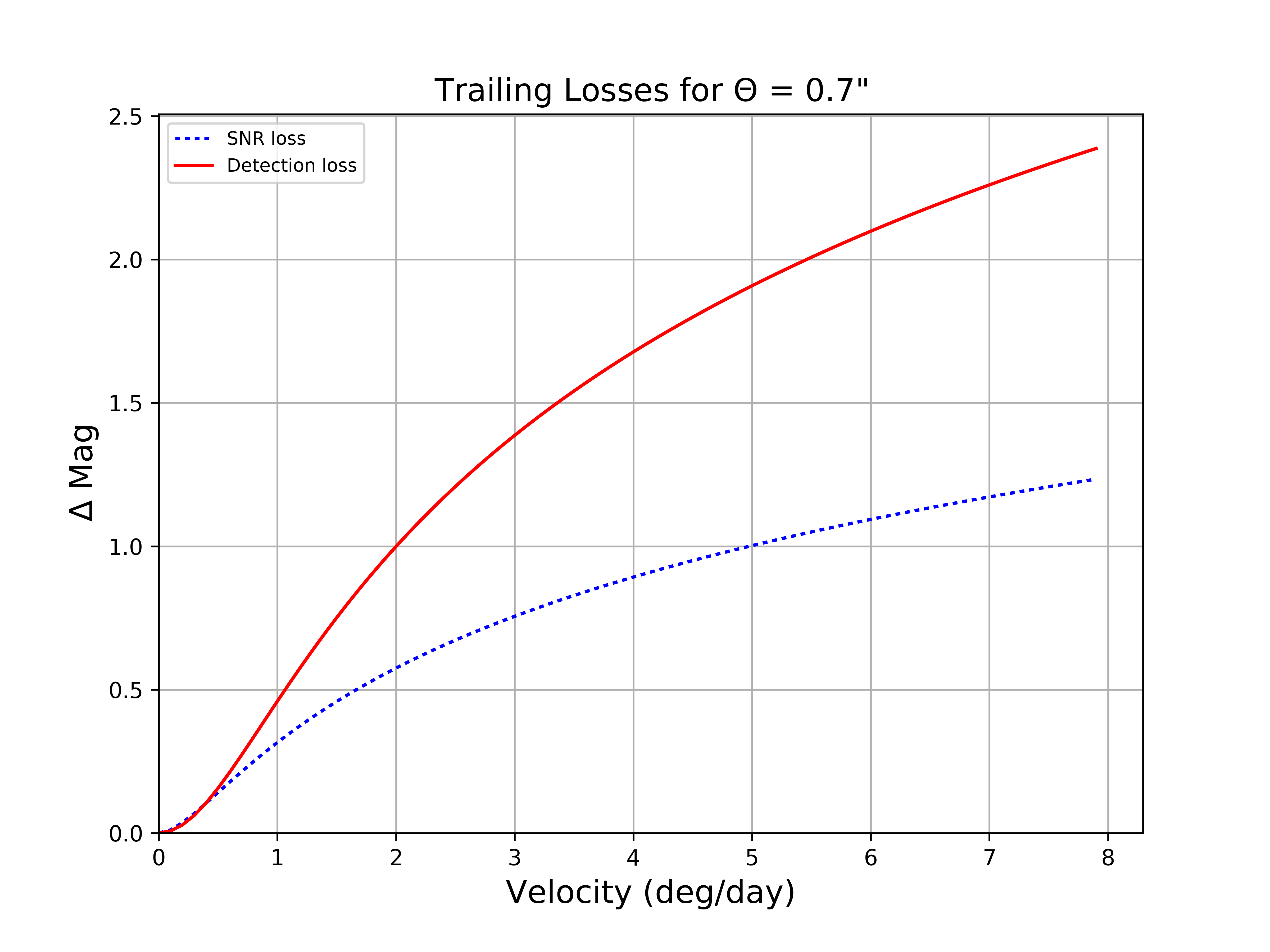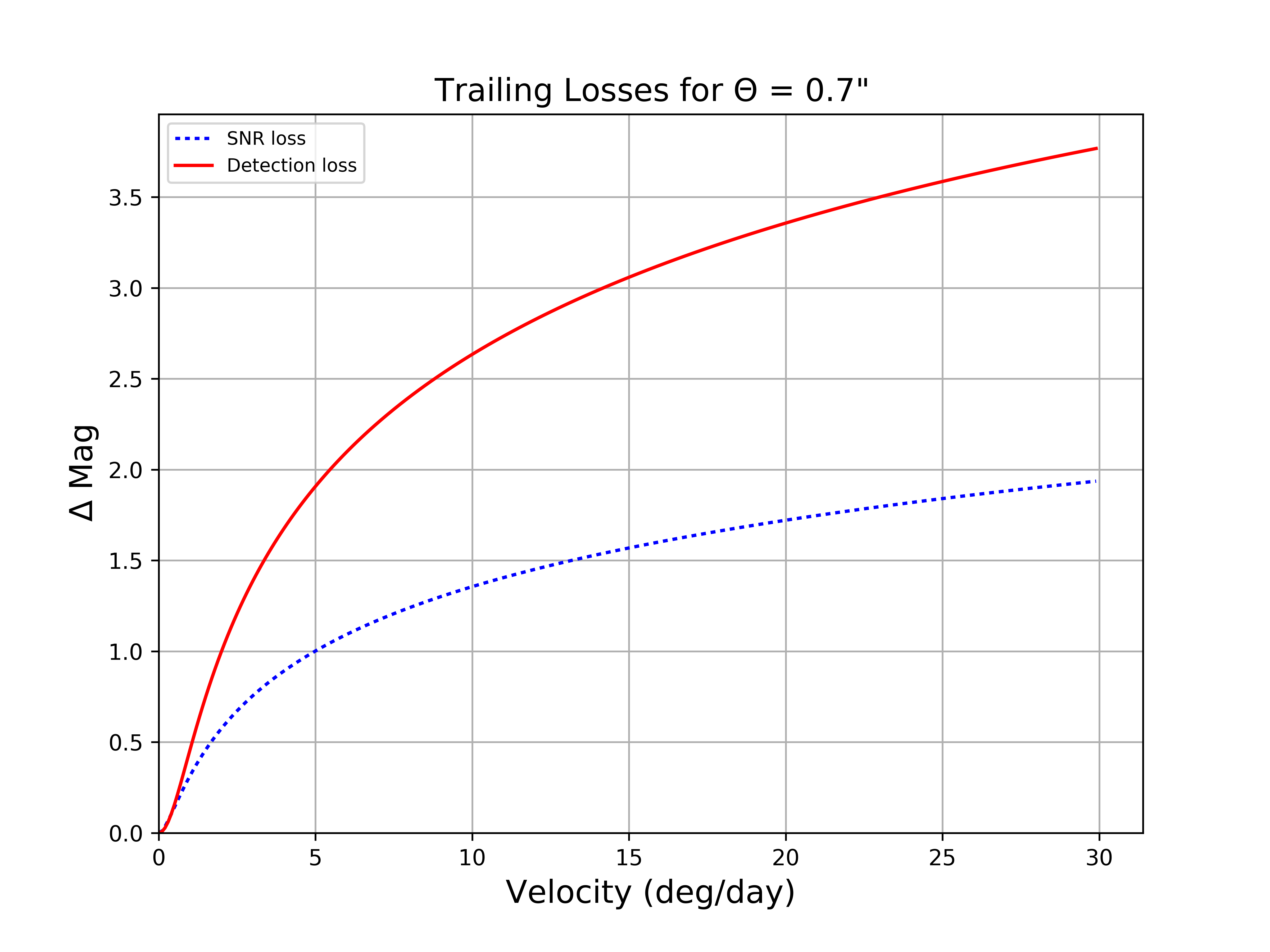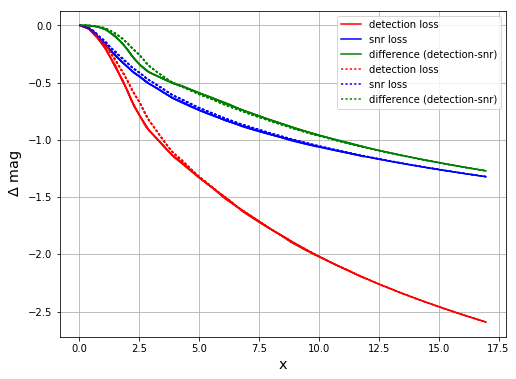# SMTN-003: Trailing Losses for Moving Objects

• Lynne Jones

Latest Revision: 2017-04-18

Solar system objects move across the image during an exposure. If the movement is significant, the signal to noise ratio of the object decreases compared to an equivalent stationary object.Figure 1 Trailing losses in 0.7” seeing for 30 second exposures. The blue dotted line (SNR loss) indicates the losses due to simply spreading the light from a moving source over more background pixels. The red line (Detection loss) indicates the losses due to detection algorithms assuming a stellar PSF instead of a trailed PSF. With additional work in the source detection software stage, detection losses can be mitigated to the level of SNR losses.Figure 2 Trailing losses in 0.7” seeing for 30 second exposures, as above, but with a wider range of velocities.Figure 3 Trailing losses as a function of “X” = velocity(deg/day) * exposure time(s) / seeing(”) / 24.0.

def trailing_losses(velocity, seeing, texp=30.):
"""Calculate detection-based and SNR-based trailing losses.

Parameters
==========
velocity : float
The velocity of the moving object, in deg/day.
seeing : float
The seeing in the image, in arcseconds.
texp : float, opt
The exposure time, in seconds.

Returns
=======
dict
dmag['trail'] and dmag['detect'] - detection and SNR losses.
"""
a_trail = 0.761
b_trail = 1.162
a_det = 0.420
b_det = 0.003
x = velocity * texp / seeing / 24.0
dmag = {}
dmag['trail'] = 1.25 * np.log10(1 + a_trail*x**2/(1+b_trail*x))
dmag['detect'] = 1.25 * np.log10(1 + a_det*x**2 / (1+b_det*x))
return dmag


Note

This technote is not yet published.

Calculating trailing losses for moving objects.